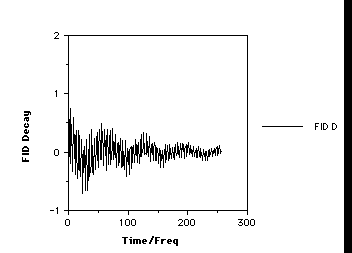# The Pulsed NMR Experiment.

Lets go back to our Precession Diagram. Our transmitter oscillator placed perpendicular to Bo generates a pulse of electromagnetic radiation producing a magnetic field B1 along the x axis. A range of frequencies +w ± 1/tp are produced, enabling resonance to be simultaneously established with all the Larmor frequencies of protons within 1/tp of +w. The phase of each set of identical resonances is rendered coherent, which "tips" the macroscopic magnetisation vector for each resonance away from Bo, by the angle q defined by;

q = g. B1.tp (6)

A value of q =90° is often referred to as a 90° pulse because of the angle the magnetisation vector is changed by, ie from the z axis into the xy plane!. In practice, values of q ~30° are often selected for practical reasons, although even 180° pulses are sometimes used. Our particular pulse stops after tp seconds, when all the various protons have an excited state population which is no longer in equilibrium with the ground state as defined by equation (5) (indeed as noted above, with a 180° pulse the equilibrium is inverted). Equilibrium is re-established via spin-lattice and spin-spin relaxation, a process which takes about 5-6 seconds for protons (cf T1and T 2 above) and which involves the return of the macroscopic vector M to the z axis, ie the "flip" angle q decays back to zero (exponentially) in about 5-6 seconds for typical protons. M for each set of different protons will have a different Larmor precession frequency, which in this example will span the range 600 Hz. As long as q is not 0, the resultant non-zero vector component of M in the direction of the receiver coil placed on the y axis induces a sinusoidal current in this receiver. Each set of distinct protons will produce a sine (or cosine) wave whose frequency matches their precession frequency and the intensity of which is related not only to the phase of the sine wave but also to the value of q at any instant (ie the magnitude of the y component of M). The signal detected in the receiver therefore resembles a collection of exponentially decaying sine waves, and is called a Free Induction Decay, or FID for short;For practical reasons, electronic circuitry in the receiver automatically subtracts the transmitter frequency +w from the measured frequencies so that only frequencies in the range 0 - 600 Hz are actually recorded. You should also note that in discussing the behaviour of the vector M, our reference point was the so called "laboratory frame of reference" in which M is "observed" to precess with frequency +w. We will simply note here that it is actually more convient to refer to a "rotating frame of reference" in which the "observer" is assumed to be also rotating around at +w (rather like riding on a carousel rather than watching it from afar) which makes the vector M appear stationary. Such descriptions are used when describing more complex NMR experiments (ie two dimensional NMR) but will not be discussed further in this particular course.